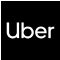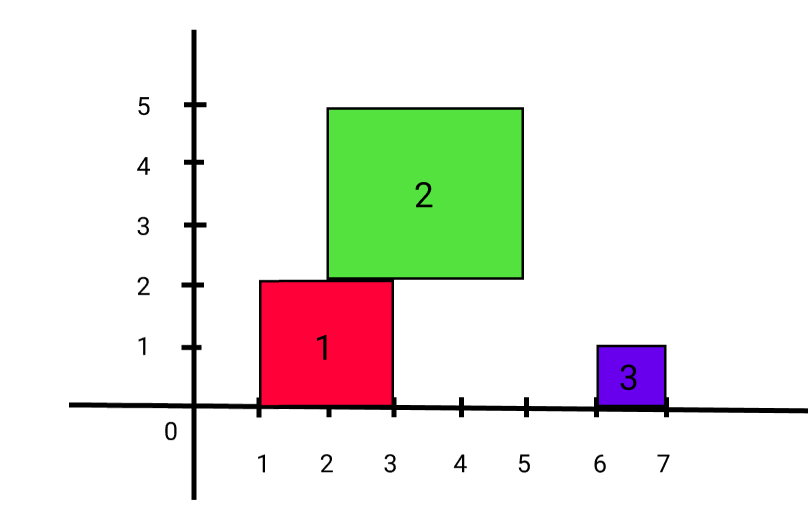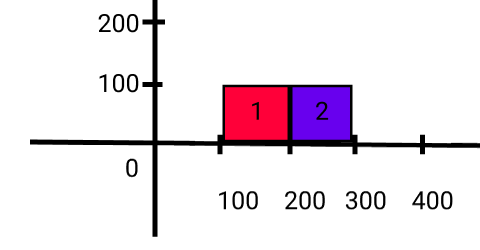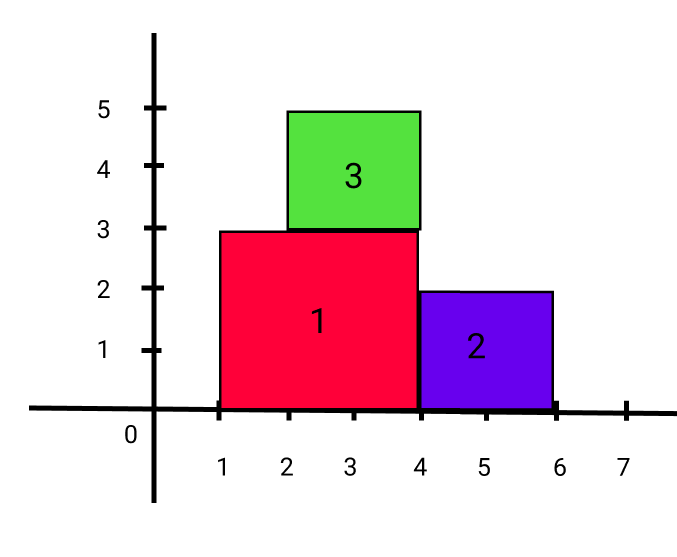New update is available. Click here to update.
Topics

# Ninja and Meteorites

Moderate0/80
Average time to solve is 25m## Problem Statement

You are a scientist observing a peculiar planet ‘NINJA_LAND’. The surface of this planet is a flat X-Y plane. ‘N’ square-shaped meteorites are falling one by one into the planet on the X-axis from space.

With your years of experience and new technology, you have been able to accurately calculate the position of the leftmost X coordinate of meteors on ‘NINJA_LAND’ along with their size.

You store this information in a 2D matrix/list ‘METEORITES’ of size ‘N’ x 2 where ‘METEORITES[i]’ denotes the leftmost ‘X’ coordinate of meteor ‘i’ and ‘METEORITES[i]’ denotes its size.

As a meteorite ‘M’ falls on ‘NINJA_LAND’ at a specific location, and if some meteorite ‘N’ is already there at that location, then the current meteorite ‘M’ will stick on top of that previous meteorite ‘N’.

Your task is to find the current highest height of any meteorite after each meteorite falls from space. Formally, return an array/list ‘HEIGHTS’ where ‘HEIGHT[i]’ denotes the highest height so far after ‘i’ meteorites have fallen on ‘NINJA_LAND’.

For example:

``````Let ‘N’ = 3 and ‘METEORITES’ = [[1, 2], [2, 3], [6, 1]].
The position and size of each meteorite are: </br>
‘Meteorite1’:  At 1 position and size of 2.
‘Meteorite2’:  At 2 position and size of 3.
‘Meteorite3’:  At 6 position and size of 1.
````````````So when the first meteorite falls maximum height till now is 2.
When the second meteorite falls maximum height till now is 5.
When the third meteorite falls maximum height till now is 5.
``````
Detailed explanation ( Input/output format, Notes, Images )
Constraints:
``````1 <= ‘T’ <= 10
1 <= ‘N’ <= 1000
1 <= ‘X’ <= 100000
1 <= ‘SIZE’ <= 10000

Where ‘T’ denotes the total number of test cases, ‘N’ represents the number of meteorites,’ X’ denotes the leftmost X-coordinate of the current meteorite and ‘SIZE’ represents the size of the current meteorite.

Time Limit: 1 second
``````
Sample Input 1:
``````1
2
100 100
200 100
``````
Sample Output 1:
``````100 100
``````

#### Explanation for Sample Output 1:

``````For sample test case 1:
````````````So, when the first meteorite falls maximum height till now is 100.
When the second meteorite falls maximum height till now is 100.
``````
Sample Input 2:
``````1
3
1 3
4 2
2 2
``````
Sample Output 2:
``````3 3 5
``````

#### Explanation for Sample Output 2:

``````For sample test case 1:
````````````So when the first meteorite falls maximum height till now is 3.
When the second meteorite falls maximum height till now is 3.
When the third meteorite falls maximum height till now is 5.
``````Console